Published: 31 December 2018

# Deep convolutional neural networks for Bearings failure predictionand temperature correlation

D. Belmiloud1
T. Benkedjouh2
M. Lachi3
A. Laggoun4
J. P. Dron5
1LDMV, MI Département, Faculté des Sciences de l’Ingnieur, 35000 Boumerdes, Algeria
1, 3, 5GRESPI, LTM Laboratory, Reims Champagne Ardenne University, 51687 Reims, France
2LMS Laboratory, EMP, 16111 Algiers, Algeria
4UR-MPE Laboratory, Boumerdes University, 35000 Boumerdes, Algeria
Corresponding Author:
D. Belmiloud
Views 825

#### Abstract

Rolling elements bearings (REBs) is one of the most sensitive components and the common failure unit in mechanical equipment. Bearings failure prognostics, which aims to achieve an effective way to handle the increasing requirements for higher reliability and in the same time reduce unnecessary costs, has been an area of extensive research. The accurate prediction of bearings Remaining Useful Life (RUL) is indispensable for safe and lifetime-optimized operations. To monitor this vital component and planning repair work, a new intelligent method based on Wavelet Packet Decomposition (WPD) and deep learning networks is proposed in this paper. Firstly, features extraction from WPD used as input data. Secondly, these selected features are fed into deep Convolutional Neural Networks (CNNs) to construct the Health Indicator (HI). This study focuses on analysing the relationships such as correlations between the HI and temperature. We develop a solution for the Connectiomics contest dataset of bearings under different operating conditions and severity of defects. The performance of the proposed method is verified by four bearing data sets collected from experimental setup called “PRONOSTIA”. The results show that the health indicator obtains fairly high monotonicity and correlation values and it is beneficial to bearing life prediction. In addition, it is experimentally demonstrated that the proposed method is able to achieve better performance than a traditional neural network based method.

## 1. Introduction

The performance degradation assessment of bearing plays an important role in various rotating machines fault to guarantee reliability in industrial processes [1-4]. Recently, studies on bearings prognostics can be seen as a problem of pattern recognition and many artificial intelligent methods, several researches have been made to develop techniques for machine health monitoring [5-7].

Cerrada et al.  gives a summary of PHM tools for bearings severity evaluation and possible failure modes, characteristics, the common available data types from different sensors, the different features and algorithms applied for prognostics and health management design.

Machine learning algorithms used in health assessment and RUL estimation depend on the features extracted. Recent developments of prognostics have focused on applying advanced techniques of signal processing to extract the robust features for constructing the health indicator . Features extraction from different signal representation such as: temporal, spectral and time-frequency [10, 11], and so on, in general do not have good monotonicity, which limits their indication in faults severity evaluation. The features extracted from sensors signals contain information about the health state of bearing. In order to produce robust and significant features, diverse original features need to be studied for show the effectiveness of the proposed approach.

Several researches have been studied the feasibility of ANN for health assessment RUL estimation. However, its accuracy is highly dependent on the neural networks structure such as a number of hidden layers, nodes and kernel function. BenAli et al.  proposed an intelligent method based on the data-driven prognostic approach by the combination of the neural networks and Weibull distribution. Rai et al.  combined the neural network approach with wavelet-based denoising method for the RUL assessment. For the improvement of the traditional neural network, CNNs have been proposed in this study for learn the features, a long short term memory based neural networks scheme was proposed by Yuan et al.  utilizing Long Short-Term Memory neural network to get good diagnosis and prediction performance in the cases of complicated operations, hybrid faults and strong noises for RUL estimation of aero-engines in the cases of complicated operations, hybrid faults and strong noises. However, it still to develop an effective approach based on historical data such as deep learning.

Recently, several learning methods has emerged called as deep learning that improved to learn higher level abstractions from the raw data [15-17], deep learning models automatically learn a feature representation from raw signal. CNNs, auto-encoders and deep belief network are the mostly known models in deep learning, and applied in many research area such as: speech recognition , image processing [19, 20], machinery condition monitoring and health assessment [21-24]. Li et al.  propose a novel deep convolutional neural network-based method for remaining useful life predictions. The aim of this study is to estimate the RUL of aero-engine units accurately. A good prognostic performance prediction is achieved with the proposed approach using raw feature selection, data pre-processing and sample preparation with time window.

In this paper, we propose a model for health assessment and RUL estimation which integrates a WPD algorithm for fast feature extraction, a nodes energy features selection of each wavelet level decomposition, and an intelligent analysis method based on the CNNs algorithm to obtain the health assessment result of bearings. An experimental verification is given by using the data from PRONOSTIA test rig . Then obtained model is applied to the acceleration signals that are collected from the bearing degradation test experiment.

This paper starts with the description of the prognostics and health management along with the proposed model in Section 2. A brief introduction of WPD for features extractions is presented in Section 3 and deep CNNs for bearing health assessment in Section 4. The proposed method is experimentally validated using bearing’s dataset in Section 5 and we demonstrated the effectiveness of the proposed approach. We close the paper with conclusions in Section 6.

## 2. The proposed model

PHM is a new concept paradigm of condition based maintenance for The improvement of system safety and reliability by monitoring the facility conditions, including the maximum of the operational availability and reduction of maintenance costs. In general, the PHM methods can be grouped in three main categories such as: data-driven approaches, model-based approaches and statistical approaches [4, 27, 2]. The PHM technologies are evolving rapidly in recent years due to the different statuses and requirements of the cases, the project involved research and development. In Fig. 1 several methods and technologies which can be regarded as the steps towards prognostics and identifying maintenance needs, to support decision making and manage operational reliability. The performance assessment lean model generally consists of three main aspects: health indicators construction, RUL estimation, and health management .

Fig. 1 illustrates the overall structure of a typical bearing PHM system. It consists of essential modules, namely, sensing, preprocessing, feature identification, and PHM. The challenge features selection for identifying bearing failure are important step in PHM and inspired great research interest. The PHM module of bearing systems in general start by anomaly detection, fault diagnosis, prognosis, and decision-making in the final step.

The failure threshold limited by using the international standards (ISO 13381-1, ISO 10816 and ISO 7919). The ISO standards limited in vibration signals energy (the root mean square RMS of vibration signal), and for different indicators are used . An illustration of RUL progression is shown in Fig. 2.

Fig. 1The flowchart of the lean model for performance assessment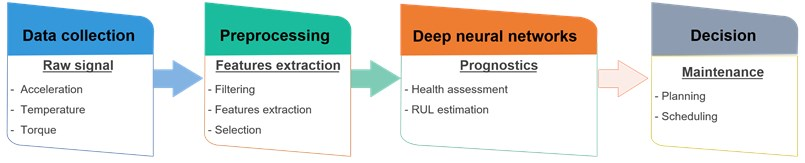Fig. 2Illustration of remaining useful life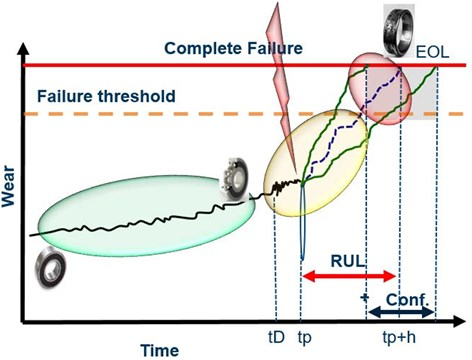## 3. Principle of WPD for feature extraction

In the proposed approach firstly, raw signals are processed by a fast, memory-saving algorithm, that is the Wavelet packet decomposition WPD nodes energy of each level is calculated. WPD is a natural extension of Multi Resolution Analysis (MRA) technique . WPD decomposes the signal using both low-frequency components and high-frequency components (Fig. 3); The flexibility of collection of abundant information for the extraction of features that combine non-stationary and stationary characteristics . The multi-level filtering process or decomposition process shown in Fig. 3.

Fig. 3Structure of two layers wavelet packet decomposition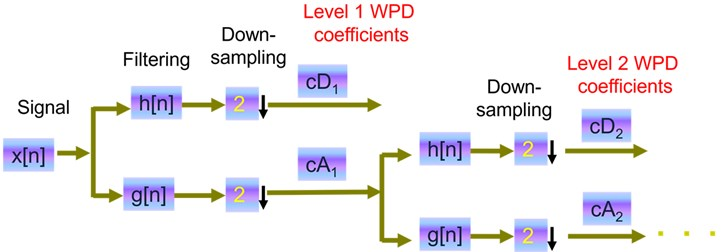The discrete signal is convolved with a low pass filter (g) and a high pass filter (h) resulting in two vectors $cA$ called the approximation coefficients and $cD$ called the details coefficients.

The process of decomposition can be repeated on the approximation vector $c{A}_{1}$ and successively on every new approximation vector $c{A}_{i}$. This concept is presented by means of a wavelet tree having $i$ levels, where $i$ is the number of iterations of the basic step. In Fig. 3 the level of decomposition equal 2.

In the current contribution, the original features are extracted from raw signals related to bearings degradations shown in the Table 1.

Table 1Feature extraction

 Approximation coefficients energy (5 levels (db6)) $RMS=\sqrt{\frac{1}{N}\sum _{i=1}^{N}‍cA\left(t{\right)}_{i}^{2}}$ Detail coefficients energy (5 levels (db6)) $RMS=\sqrt{\frac{1}{N}\sum _{i=1}^{N}‍cD\left(t{\right)}_{i}^{2}}$ Signal to noise ratio (SNR) $SNR=\frac{{P}_{signal}}{{P}_{noise}},$ Peak signal to noise ratio (PSNR) $PSNR=10.log\left(\frac{MA{X}_{x}^{2}}{MSE}\right)$

Fig. 4Sensors measurement of normal conditions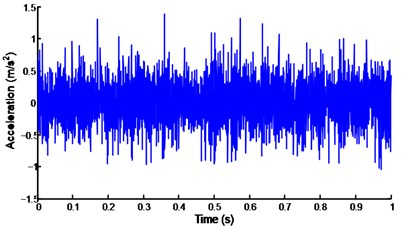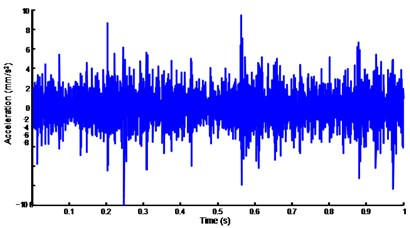In Fig. 6 shown that there are a few helpful to consider when looking at the multitude of time frequency transform. It is helpful to go over a couple of these with another statistical parameters, because they are heavily utilized in condition monitoring. Increasing sample rate does not add information to frequency peaks of interest. The nodes energy coefficients evolution at each level shown in 7 is equal to the length of raw signals after decomposition using (db6), the WPD coefficients can retain more fault severity information, and hence can be extracted more distinguishing statistical features for RUL estimation.

In Fig. 8 shown that the monitoring temperature on the surface of the bearing housings with temperature probe (PT100) has been used in this paper. Under different operating conditions of load and speed, the temperature of a bearing is monitored for changes that can indicate defect in bearing elements. In this study the experimental has shown that temperature is a good indicator of load, speed or lubrication than of bearing condition.

Temperature monitoring techniques may be helpful in preventing machine breakdown. However, bearing defects have not been found to cause an appreciable increase in temperature until the damage has reached a severe state.

In Fig. 8 shown that the monitoring temperature technique for bearing prognostics becomes far more complicated when it is subject to changeable operating condition. In most situations, varying operating conditions refer to variable loading conditions since it is the major source of contribution to the energy of the measured vibration signal.

Fig. 6Time frequency transform for tested bearing (6h50)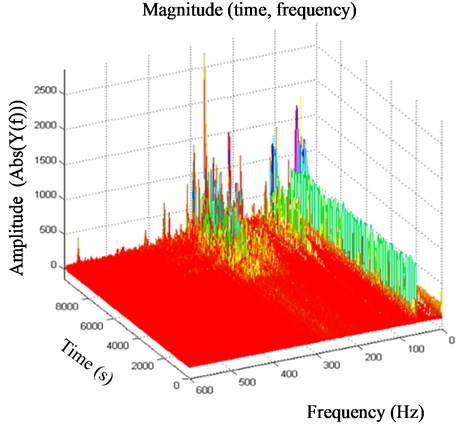Fig. 7Wavelet packet decomposition for tested bearing (6h50)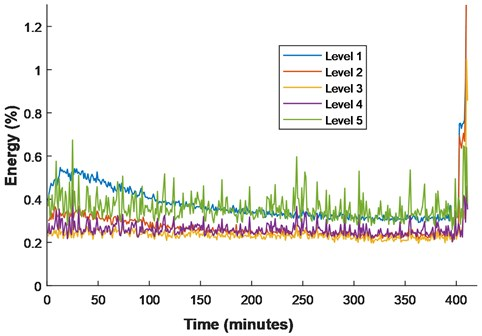Fig. 8Temperature evolution for tested bearing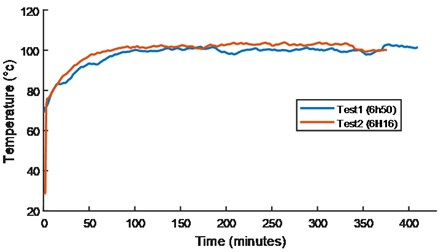a)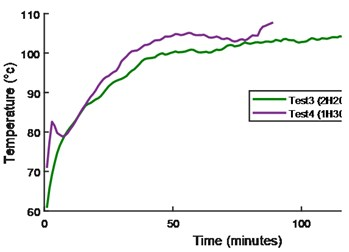b)

## 4. Deep CNNs-Based bearings health assessment

This section presents the architecture of deep learning CNNs for health assessment and RUL estimation from different sensor signals. The inputs features are extracted from different operating condition history. The output values are the RUL of bearings at corresponding lifetime. In honor Yann LeCun  an early pioneer of CNNs and the first to reduced them to practice by training them with gradient descent. For image processing, which is featured by two key properties: spatially shared weights and spatial pooling.

Fig. 9Illustrations of the proposed CNNs for bearing prognostics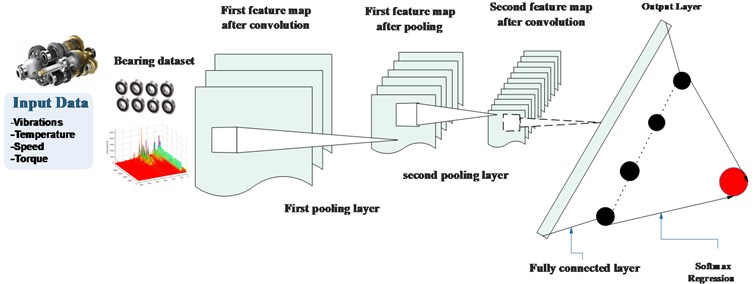The deep learning algorithms are machine learning technique based on distributed representations. Using structures composed of multiple non-linear transformations deep learning attempts to learn high-level features in data. The frequently used models are CNNs and Deep Belief Network (DBN).

CNNs is also a type of feedforward neural network which is composed of alternating convolutional and subsampling layer [32, 33]. CNNs are designed to use minimal amounts of preprocessing, which is the main difference compared to other deep architectures.

Firstly, we assume that the input sequential data is $y=\left[{y}_{1},...{y}_{n}\right]$ that $n$ is the length of the sequence and ${y}_{i}\in {R}^{d}$ at each time step. Convolution: the dot product between a filter vector $u\in {R}^{m}d$ and an concatenation vector representation ${y}_{i:i+m-1}$ defines the convolution operation as follows:

1
${c}_{i}=\phi \left({u}^{T}{y}_{i:i+m-1}+b\right),$

where $b$ and $\phi$ denotes bias term and non-linear activation function, respectively. ${y}_{i:i+m-1}$ is a $m$-length window starting from the $i$th time step, which is described as:

2
${y}_{i:i+m-1}={y}_{i}\oplus {y}_{i+1}\oplus ...\oplus {y}_{i+m-1}.$

As defined in Eq. (2), the output scale ${c}_{i}$ can be regarded as the activation of the filter $u$ on the corresponding subsequence ${y}_{i:i+m-1}$. By sliding the filtering window from the beginning time step to the ending time step, a feature map as a vector can be given as follows:

3
${c}_{j}=\left[{c}_{1},{c}_{2},...,{c}_{l-m+1}\right],$

where the index $j$ represents the $j$th filter. It corresponds to multi-windows as:

$\left\{{y}_{1:m},{x}_{2:m+1},...,{y}_{l-m+1:l}\right\}$. Max-pooling: able to reduce the length of the feature map, which can minimize the number of model parameters. The hyper-parameter of pooling layer is pooling length denoted as $s$. MAX operation is taking a max over the s consecutive values in feature map ${c}_{j}$.

Then, the compressed feature vector can be obtained as:

4
$h=\left[{h}_{1},{h}_{2},...,{h}_{\frac{l-m}{s}+1}\right],$

where ${h}_{j}=\mathrm{m}\mathrm{a}\mathrm{x}\left({c}_{\left(j-1\right)s},{c}_{\left(j-1\right)s+1},...,{c}_{\left(js-1}\right)$. Then, via the two layers: convolution and max-pooling ones, the fully connected layers and a softmax are usually added to make predictions by the top layers.

The proposed method diagram for the bearings health assessment and RUL estimation shown in Fig. 10. The method is decomposed into two main phases the preprocessing data and training data using deep CNNs. the bearing dataset prepared for the training by computing the features. All the layers use activation functions $\mathrm{t}\mathrm{a}\mathrm{n}h$. Then training and testing datasets are prepared for deep CNNs. In the second phase, which is achieved on-line, deals with the utilization of the model generated continuously to assess the health state of the bearing and RUL prediction.

Fig. 10Flow chart of the proposed method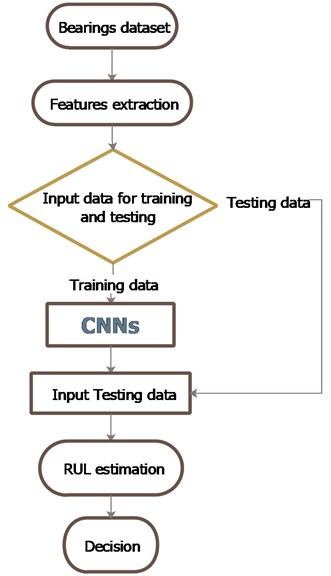## 5. Application: performance assessment of REBs

### 5.1. Experimental system

An accelerated bearing life test platform called PRONOSTIA  (Fig. 11) is used in this section to verify the prognostics of proposed method. PRONOSTIA is a laboratory experimental platform dedicated to test, verify and validate developed methods related to bearing health assessment, diagnostic and prognostic. In the following of the paper, a set of four experiments consisting of four degraded bearings has been utilized. The four data shown in Table 2 for the different loading and speed motor.

Table 2Bearings dataset from PRONOSTIA experimental setup

 Tests duration Loading (N) Speed (RPM) h50 4000 1800 h16 4000 1800 h20 6000 1500 h30 8000 1500

Fig. 11PRONOSTIA platform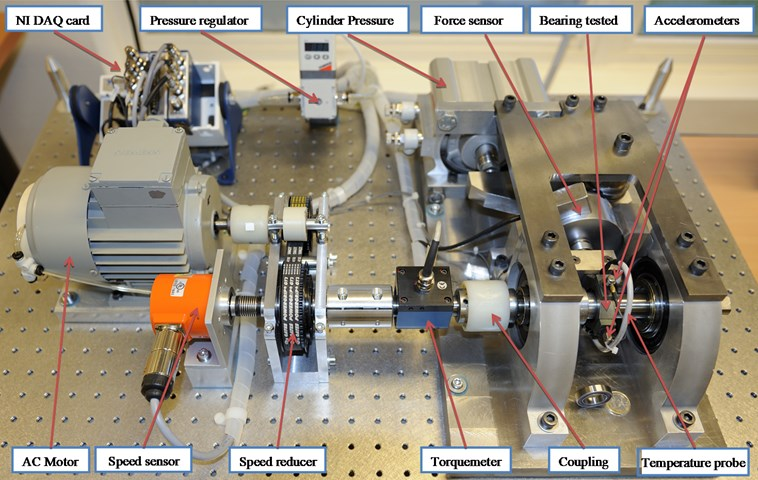The PRONOSTIA experimental setup composed of two main parts: The first part related to the speed control and a second part to load profiles generation. The speed control part is composed of electric motor, shaft, a set of bearings. The power developed by motor equal to 1.2 kW and its speed varies between 0 to 6000 rpm. The second part of PRONOSTIA contain a hydraulic jack connected to a lever arm used to create different loads on the tested bearing mounted on the platform.

A pair of ball bearings is mounted on one end of the shaft to serve as the guide bearings and a NSK6307DU roller ball bearing is mounted on the other end to serve as the test bearing. The transmission of the movement between the motor and the shaft drive is done by a rub belt. Two accelerometers (DYTRAN3035B) mounted horizontally and vertically on the housing of the tested bearing to pick up the horizontal and the vertical accelerations (Table 3). In addition, the monitoring system includes one temperature probe and a torque sensor (Fig. 11). The sensors are connected to a data acquisition card.

The data acquisition software is programmed by using a LabView interface. Each record is stored in a matrix format where the following parameters are defined: the time, the horizontal acceleration, the vertical acceleration, the temperature, the speed and the torque.

Table 3Experimental data acquisition

 Measurement Type Acceleration (DYTRAN3035B) Temperature $\mathrm{P}\mathrm{T}100$ Torque (DR2269) Force (AEPC2S) Acquisition card (NIDAQCard-9174) Sampling frequency 25600 Hz Motor speed 6000 rpm, 1.2 kW

With this experimental platform, several types of profile can be created by varying the operating conditions (speed and load). The bearing’s behavior is captured during its whole degradation process by using the dedicated sensors. The tested bearing has the characteristics shown in Table 4.

Table 4Tested bearing

 Bearing type Ø Outside Ø Inside Balls Angle Ø Ball NSK6804DD 32 mm 20 mm 8 $\alpha =$ 15° 3.5 mm

### 5.2. Results and discussion

In order to identify different working conditions monitoring of REBs, the proposed fault prognostics method based on CNNs and WPD is performed. The initial operator $P$ and $U$ with $M=$ 4 and $N=$ 4 are calculated. In this experimentation, each signal was decomposed to level 6, then eight subband coefficients were obtained. The classification errors are reported in Table 5. The PRONOSTIA experimental setup system are shown in Fig. 11. A working cycle of vibration for different loading and speed motor are saved, which include all the data for each REBs. The classification accuracies of REBs on the PRONOSTIA experimental setup were calculated and reported in Table 5. Also, the classification results obtained by using the features extracted from time domain (Fig. 4 and 5) and from time-frequency domain (Fig. 6).

Fig. 12Health indicator for the tested bearings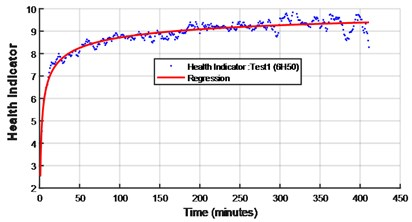a)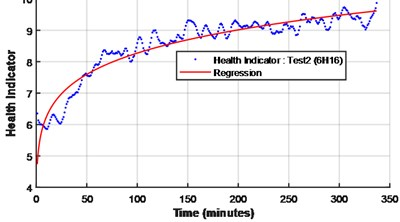b)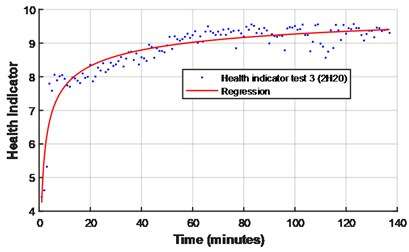c)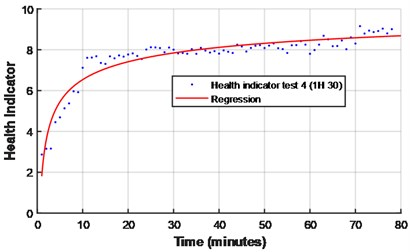d)

From Table 5, we can find a considerable improvement in the classification accuracies compared to the results obtained by using the WPD based fault prognostics method with different loading of the classifier. Another popular metric for the performances evaluation method is the Root Mean Square Error (RMSE). The RMSE of RUL estimation is employed as a performance measure shown in Fig. 13.

From the above four experiments, we can find that the classification accuracies obtained by using the CNNs and WPD are higher than those obtained by using WPT and statistical parameters-based fault prognostics method .

The regression results are presented in Table 5 in terms of the factors of determination ${R}^{2}$ for the different training models. The $R$ values, indicating the fraction of the total variance that could be explained by the model, are very high. From the results, it is seen that all the predictors perform very well. The objective is to apply the best power fit on the degradation model obtained by Eq. (6):

5
$f\left(t\right)=a.{t}^{b}+c,$
6
$RUL\left(t\right)={t}_{final}-H{I}^{-1}\left(t\right),$

where ${t}_{final}$ is the time when the fault occurs and $H{I}^{-1}$ the inverse of the health indicator $HI\left(t\right)$ used to get the current cycle or time $\left(t\right)$. The validation of these results shown in Table 5 by computing of the sum square error (SSE), ${R}^{2}$ RMSE.

Fig. 13Comparison between scoring function and error value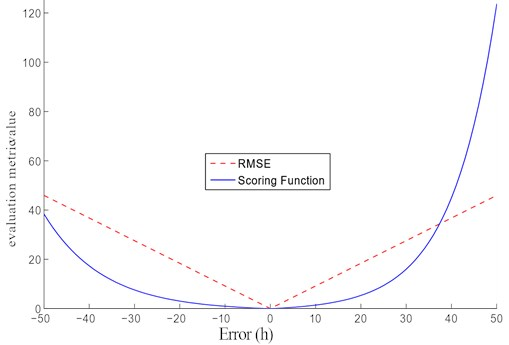Table 5Prediction performance

 Bearings RMSE ${R}^{2}$ Regression function H50 0.2285 0.9451 $f\left(t\right)=-8.058*{t}^{-0.3166}+10.59$ H16 0.3019 0.9342 $f\left(t\right)=-8.335*{t}^{-0.3096}+10.42$ H20 0.2996 0.9258 $f\left(t\right)=-6.297*{t}^{-0.3458}+10.55$ H30 0.4168 0.8961 $f\left(t\right)=-9.237*{t}^{-0.3117}+11.04$

The RUL estimation is the distance between the current time and the time for which the regression model given in Eq. (5). The threshold or the acceptable limit of the vibration magnitude  of each degradation in bearings, corresponds to the end of each experiment. The power fitting of the smoothed health indicator is shown in Fig. 12.

### 5.3. Temperature correlation

The correlation between temperature and health indicator given a result shown in Fig. 14. More advanced prognostics interested on performance degradation assessment, so that failures can be predicted and prevented using the temperature and vibrations signal obtained in this study by CNNs for regression. As soon as, the concept of correlation between the two indicators for accurately assessing the bearing performance degradation is a critical step toward realizing an online tool condition monitoring platform. The results of its application for performance degradation assessment show that this indicator can reflect effectively the performance of degradation process.

It has been shown that the health indicator obtained by vibrations measurements given the same monotonicity compared to temperature measurements.

Fig. 14Temperature and health indicator obtained by CNNs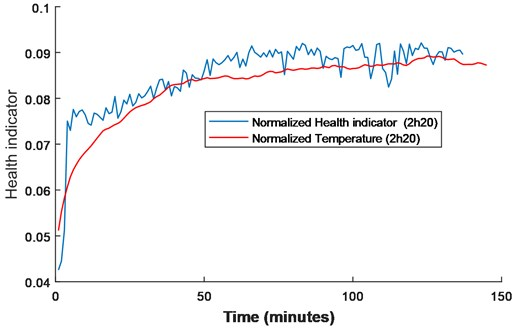Fig. 15Temperature and health indicator obtained by CNNs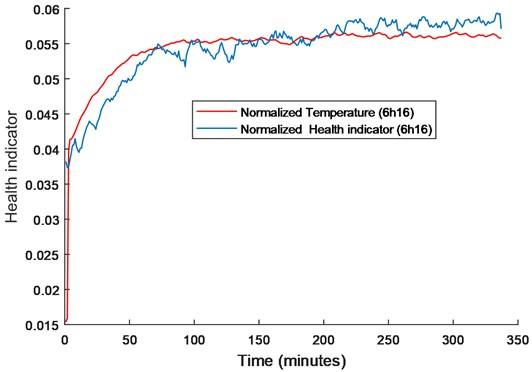## 6. Conclusions

In this paper, a novel fault prognostics method for REBs based on WPD and CNNs have been proposed. The model develops an effective RUL prediction method that addresses multiple challenges in complex system prognostics where many parameters are unknown. In order to investigate the effectiveness for practical applications, the proposed method is tested by using PRONOSTIA experimental setup. The test results show that the proposed method can achieve a higher classification accuracy rate than the result obtained by using wavelet packet transform based prognostics method. The good experimental results obtained method, further architecture optimization is still necessary. Deep learning methods generally suffer from high computing load, and that will be focused on in further research. In this study Temperature and vibration analysis are two dominating condition monitoring techniques applied to bearing failures prognostics. The temperature HI has reliable revealed the all change of the bearings condition monitoring and rendering the diagnosis procedure less complicated. For the proposed algorithm evaluation, we examined the performance on four bearing’s data sets. The experimental results of bearing data sets demonstrate the superiority of the proposed CNNs model to other fault-prognostics methods. The proposed approach achieves a high degree of accuracy.

#### Cited by

A Robust Health Prognostics Technique for Failure Diagnosis and the Remaining Useful Lifetime Predictions of Bearings in Electric Motors
A machine-learning based data-oriented pipeline for Prognosis and Health Management Systems
Application of Time-Frequency Analysis in Rotating Machinery Fault Diagnosis
Fault Size Estimation of Bearings Using Multiple Decomposition Techniques with Artificial Neural Network
Fault Diagnosis of Rotating Machinery Based on Evolutionary Convolutional Neural Network
Artificial intelligence in prognostics and health management of engineering systems
Deep learning-based algorithm for vehicle detection in intelligent transportation systems
Challenges and Opportunities of AI-Enabled Monitoring, Diagnosis & Prognosis: A Review
A comprehensive review on convolutional neural network in machine fault diagnosis
Supervised Health Stage Prediction Using Convolutional Neural Networks for Bearing Wear

15 January 2018
Accepted
15 July 2018
Published
31 December 2018
SUBJECTS
Fault diagnosis based on vibration signal analysis
Keywords
bearing
WPD
features extraction
CNNs
prognostic
RUL
temperature
Author Contributions

Dalila Belmiloud, Tarak Benkedjouh and Mohamed Lachi conceived of the presented idea for the bearings prognostics and correlation with temperature using artificial intelligence techniques. Dalila Belmiloud developed the theory of deep learning and performed the computations with the decision making of the score correlation between health indicator and temperature evolution. Mohamed Lachi and Ali Laggoun verified the analytical methods from the different stages of looping and convolution. Tarak Benkedjouh encouraged Dalila Belmiloud to investigate and supervised the findings of this work. All authors discussed the results and contributed to the final manuscript and the different steps for writing the documents. Dalila Belmiloud developed the theoretical formalism, performed the analytic calculations and performed the numerical simulations from experimental data. Both Dalila Belmiloud and Tarak Benkedjouh authors contributed to the final version of the manuscript. Tarak Benkedjouh supervised the project. Dalila Belmiloud, Tarak Benkedjouh, Mohamed Lachi, Ali Laggoun, Jean Paul Dron conceived and planned the experiments and contributed to sample preparation and the interpretation of the results. Dalila Belmiloud took the lead in writing the manuscript. All authors provided critical feedback and helped shape the research, analysis and manuscript. Dalila Belmiloud and Tarak Benkedjouh designed the model and the computational framework and analyzed the data from data acquisition, features extraction and remaining useful life estimation. Dalila Belmiloud and Mohamed Lachi carried out the implementation and wrote the manuscript with input from all authors. Dalila Belmiloud designed and derived the models and analyzed the data. Tarak Benkedjouh assisted all measurements from PRONOSTIA experimental setup. Dalila Belmiloud and Tarak Benkedjouh worked out almost all of the technical details, and performed the numerical implementation of the suggested experiment. Dalila Belmiloud, Tarak Benkedjouh, Mohamed Lachi and Ali Laggoun contributed to the design and implementation of the research with future scope and listing the efficiency when compared with other methods and the future scope of the work implemented on this paper and the limitation of the proposed solution in practice, to the analysis of the results and to the writing of the manuscript.